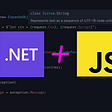# C# Heresy: Converting Expression Trees to Source Code

Over the past year, I’ve found myself in the strange position of trying to decompile C#. And not just decompile C# into something that looks more-or-less like source code — I’ve been trying to decompile C# into valid source code that I can then compile and use without modification.

In this article, I want to talk a bit about an interesting technical problem I ran into during this journey. As far as I know, nobody has described or solved this problem yet (probably because nobody has needed to compile decompiled C#), so you’re about to gain some HYPER RARE knowledge.

Today’s question is: how do we convert C# expression trees into C# source code?

# A Very Short Introduction to Expression Trees

While C# is a statically-typed compiled language, it has powerful support for runtime code generation through a feature called expression trees. Expression trees are runtime constructs that represent C# code, and they can be compiled into anonymous functions at runtime. In a way, it’s a lot like `eval` in Javascript or Python-- except it's type-safe in the same way as C# code, just as fast as C# code, and is much more secure than the unbounded execution provided in other languages.

As an example, the code `x = 1 + 2` can be represented by the expression:

`Expression.Assign(Expression.Parameter(typeof(int), "x"),                   Expression.Add(                      Expression.Constant(1),                      Expression.Constant(2))                 )`

As you can see, expression trees are incredibly verbose compared to source code, but the basic structure of the code is the same. If you’re familiar with abstract syntax trees, I think it’s fair to say that expression trees are just a type of abstract syntax tree that you can manipulate and compile at runtime.

# Literally Why?

It’s easy enough to convert an expression tree into something that looks kind of like C# source code, since the structure is so similar. For any debugging purpose, that’s sufficient. So why would anyone need to convert expression trees into actual C# code, given that expression trees are runtime constructs and source code is only useful at compile time?

Admittedly, the circumstances for this investigation of mine are unusual. In the game engine I’ve been working on, complex ingame scenarios are described by a scripting language that, at runtime, is parsed and converted into lambdas or state machines using expression trees. However, some platforms (such as iOS and Switch) prohibit runtime code generation, preventing expression trees from being compiled.

While all the code pertaining to these complex ingame scenarios is technically present at compile time (in the form of this custom scripting language), it’s only “compiled” at runtime. This means that, if I could find a way to convert the runtime expression trees backwards into C# source code, I could use that source code as a replacement for the expression trees and enable the engine for use on iOS and Switch.

Again, this is an odd use-case, but there might be similar considerations about replacing expression trees with source code in, say, code generated for an ORM. In fact, I originally thought of this idea when reading about source generators, and there may be good reason for someone to write a source generator that creates expression trees and then outputs them as source code. Maybe I’ll find a proper reason to do this just to retroactively justify the amount of time I’ve spent on it.

# The NUMBER ONE reason why your code FAILS!

There are several solutions to expression tree printing in C#, but of all of them that I’ve looked at, I’ve found that they all struggle heavily with the same problem: block expressions.

C# doesn’t have generalized block syntax, so let’s quickly define some terms. A block is a list of parameters that are local to the block combined with a list of sequential statements that constitute the block. One of the few cases where C# does have block syntax is if/else statements:

`int y;y = 2;if (someCondition){       //this is a block    int x;    x = -1;    x = x + 6;    y = x + 2;}`

We can say that the block has just `int x` as a local parameter, and has three statements `x=-1`, `x=x+6`, and `y=x+2`. As far as expression trees go, we should consider declarations (`int x`) and definitions (`x = -1`) separately; joined declaration-definitions are just syntactic sugar.

C# expression trees are a bit strange in that every block also has a value. In the same way that `x` has a value (first of -1, then of 5) and `x + 2` has a value (of 7) and `y = x + 2` has a value (also of 7), the block itself has a value, which is the value of the last statement in the block. In expressions, the value of the block above would be the value of `y = x + 2`, which is 7.

If a block has a value, then, naturally, you can use it like a value. For example, in C# expressions, you could write the following:

`//This is not C# source. It is a source equivalent of what expression trees look like.int y;y = 2;int z;z = 4 + block({    int x;    x = -1;    x = x + 6;    y = x + 2;})`

Since the value of the block is 7, `z` gets set to 11.

Of course, we can arbitrarily nest these:

`//This is not C# source. It is a source equivalent of what expression trees look like.int y;y = 2;int a;a = 1 + block({    //Outer block    int z;    z = 4 + block({        //Inner block        int x;        x = -1;        x = x + 6;        y = x + 2;    });    z + 3;});`

In this example, `z` gets assigned to 11, and then the outer block has the value `11+3=14`, so `a` gets assigned to `1+14=15`.

In my experience, a lot of expression trees tend to look like this, because it’s so easy to generate expression trees that looks like this. And that’s perfectly fine — until you try to turn it into C# source code. There’s no easy way to convert this code into C# source code, because C# source code doesn’t support blocks everywhere. To make this valid C#, we have to get rid of the blocks, or at least put them somewhere where they aren’t in the middle of addition operations.

So how do we solve this problem? Let’s take a closer look at the example above. Intuitively, if the inner block were to contain only the statement `y = x + 2`, then it would be acceptable C# source code if we just wrote `z = 4 + (y = x + 2)`. And if we want to make the inner block only consist of that last statement, then all we have to do is move the other parameters and statements into the outer block:

`//This is not C# source. It is a source equivalent of what expressions look like.int y;y = 2;int a;a = 1 + block({    //Outer block    int z;    int x;    x = -1;    x = x + 6;    z = 4 + (y = x + 2);    z + 3;});`

Now let’s do it again:

`//This is valid C# source :)int y;y = 2;int a;int z;int x;x = -1;x = x + 6;z = 4 + (y = x + 2);a = 1 + (z + 3);`

At a basic level, we can solve the problems with block expressions by moving all the non-last statements in the block outside of the block, and then treating the last statement as a simple expression. I call this process linearization because, intuitively, it’s about taking all the deeply nested statements in the expression tree and putting them in one neat single-file line.

# Linearization

I define a linearized expression as any of the following three possibilities:

1. an expression that can participate in basic C# value operations, ie. that can take the place of X in `a = 1 + X`;
2. a block expression whose statements satisfy (1) or (3) but are not block expressions, and whose last statement (constituting the value of the block) must satisfy (1);
3. An expression with a value of type void, whose children are all linearized.

We can put aside (3) for now, as it’s primarily about other “block-like” expressions such as if/else and try/catch.

To see why this definition is useful, let’s do some induction. Let’s say we have an expression tree that looks like `z = A + B`, where A and B are both highly complex block expressions, and a function `Expression Linearize(Expression)`. In order to linearize `z = A + B`, we first recursively linearize A and B into LA and LB. Observe that, since `z = A + B` always requires that A and B have non-void values, LA and LB will satisfy either (1) or (2).

First, let’s assume that both satisfy (1). In that case, we can simply write `z = LA + LB`, according to the definition of (1).

For the more general case, let’s assume that both satisfy (2), so both are block expressions. We need to move their parameters and statements into the outer scope and use their last statements in `z = A' + B'`:

`var blockParameters = new List<ParameterExpression>();var blockStatements = new List<Expression>();//Recursively linearize A and Bvar LA = Linearize(A);var LB = Linearize(B);​//Combine the parameters of both LA and LBblockParameters.AddRange(LA.Parameters);blockParameters.AddRange(LB.Parameters);​//Move all but the last statements of LA and LB into the outer scope// (Python syntax for brevity)blockStatements.AddRange(LA.Statements[:-1]);blockStatements.AddRange(LB.Statements[:-1]);​//Use the last statements of LA and LB in z = A' + B'// (Python syntax for brevity)var assignExpression = Assign(z, Add(LA.Statements[-1], LB.Statements[-1]));blockStatements.Add(assignExpression);​//Construct a single block, satisfying (2), with all the statements and parametersreturn new BlockExpression(blockParameters, blockStatements);`

In the fully general case, it’s possible that one satisfies (1) and the other satisfies (2), but that’s not difficult to resolve using this general approach.

This code explicitly handles our `z = A + B` case. We can use the same process to create a function that generally linearizes any expression tree by recursively calling into itself. But before we do that, let's talk a bit about expression visitors.

# Expression Visitors

In C#, whenever you’re performing some kind of generalized operation over an expression tree, you probably want to use an expression visitor. The builtin C# class `ExpressionVisitor` uses the visitor pattern to visit all the expressions that are children or descendants of a given expression tree. By inheriting this class and overriding functions specific to the expression types we're concerned with, we can modify the expression tree or perform some statistical analysis on it.

Here’s an example: let’s say we wanted to create a visitor that increases any integer constants by 1, so the expression `(1 + (2 * 3))` would turn into `(2 + (3 * 4))`. In this case, we only need to modify the handling for ConstantExpression, so we could write:

`class ModifyConstVisitor : ExpressionVisitor {    protected override Expression VisitConstant(ConstantExpression node) {        if (node.Value is int i)            return Expression.Constant(i + 1);        else            //No changes            return node;    }}​var modifiedExpression = new ModifyConstVisitor().Visit(myExpression);`

The base class handling in ExpressionVisitor.Visit() receives an expression tree of any type, then delegates it to the appropriate type-specific method. Its default handling for the type-specific method is simply to call Visit() on all the children of that expression tree and reconstruct the expression tree. As a result, even if the constants in `myExpression` are not at the top level-- like the 2 and 3 in `(1 + (2 * 3))`-- they get visited by ExpressionVisitor.

`//ExpressionVisitor handling (not actual code, but close enough)protected virtual Expression VisitBinary(BinaryExpression node) =>     Expression.MakeBinary(node.NodeType, Visit(node.Left), Visit(node.Right));`

Our linearization process can also be described as an expression visitor. To handle an expression, we recursively linearize its children, then reconstruct the expression using the last statements from the block expressions that result. The specific process for doing this reconstruction step, which looks like `Expression.MakeBinary` or `Expression.Constant`, will differ by the expression type, but the basic pattern of linearizing children and combining their last statements will be similar.

# Putting it All Together

To encode this pattern, we’ll have a separate function that generically handles combing the last statements of children, and each of the VisitX overrides will call into it.

`private Expression Linearize(Func<Expression[], Expression> combiner, params Expression?[] pieces) {    //Linearize all provided children    Expression?[] linearized = pieces.Select(Visit).ToArray();    //If the results are only (1)s, then we can trivially combine them    if (!linearized.Any(ex => ex is BlockExpression))        return combiner(linearized);    //Otherwise, we create a new block expression that combines all of the inner block expressions    var parameters = new List<ParameterExpression>();    var statements = new List<Expression>();    var lastStatements = new Expression?[linearized.Length];for (int i = 0; i < linearized.Length; ++i)        //As far as I know, there is no case where this can have a type of void (rule 3).        if (linearized[i] is BlockExpression bex) {            //Rule 2            parameters.AddRange(bex.Variables);            statements.AddRange(               bex.Expressions.Take(bex.Expressions.Count - 1));            lastStatements[i] =                            bex.Expressions[bex.Expressions.Count - 1];        } else            //Rule 1            lastStatements[i] = linearized[i];//Add the final statement    statements.Add(combiner(lastStatements));    return Ex.Block(parameters, statements);}`

This is our generic function. If we wanted to linearize `A + B`, we could call `Linearize(exs => Ex.Add(exs, exs), A, B)`. Each expression type will call Linearize differently, but the basic calls look something like this:

`//A BinaryExpression is composed of a type, a left expression, and a right expression.//The two expressions need to be linearized, and then the results // (the last expressions of the resulting blocks if (2)) can be passed to MakeBinary.//In the case of A + B, node.Left is A, node.Right is B, and node.NodeType is ExpressionType.Add.protected override Expression VisitBinary(BinaryExpression node) =>    Linearize(exs => Ex.MakeBinary(node.NodeType, exs, exs), node.Left, node.Right);protected override Expression VisitUnary(UnaryExpression node) =>    Linearize(exs => Ex.MakeUnary(node.NodeType, exs, node.Type), node.Operand);//...We also need overrides for all the other expression types`

The rest of the expression types have type-specific handling, but in general, most of them will call into Linearize and then reconstruct the expression using the linearized values.

# Bonus: Linearizing If/Else

C# expression trees don’t differentiate between if/else and ternaries — they’re both just `ConditionalExpression`. However, in C# source, we have to deal with several differing restrictions:

• An if/else statement always has a “type” of void.
• A ternary never has a “type” of void.
• The branches of an if/else statement can be blocks.
• The branches of a ternary cannot be blocks.
• For both an if/else and a ternary, the condition cannot be a block.

This raises a big problem. What happens if we come across a ConditionalExpression which has a non-void type, but whose branches are blocks? To convert it to C# source, we have to treat it as a ternary due to its type, but there are issues linearizing it. For example:

`//This is not C# source. It is a source equivalent of what expressions look like.int y;int x;y = 4;x = (y > 2) ?        block({            int a;            a = 4;            a + 7;        }) :        block({            int b;            b = LaunchNukes();            b - 9;        });`

If we took the straightforward approach to linearizing this, we would end up with the following:

`int y;int x;y = 4;int a;a = 4;int b;b = LaunchNukes();x = (y > 2) ?         (a + 7) :        (b - 9);`

This code is incorrect — we end up calling `LaunchNukes()` even though we never should have entered the false branch. The statements belonging to each branch only get executed if that branch is entered, so it's incorrect to execute them outside the branch. How can we avoid executing the statements outside of the branch, but also maintain the C# limitations on ternaries?

The answer is that we can’t — but we don’t need to. The linearization rules don’t say anything about preserving ternaries. Instead, let’s take advantage of rules (2) and (3). Since the branches are blocks, we have to use an if/else statement — there’s no way around that. But as long as we put that if/else inside another block that has has the correct type of int, there’s no problem. We can do this by creating a variable outside the if/else statement and writing to it in both branches:

`//Mostly linearizedint y;int x;y = 4;x = block({    int flatTernary;    if (y > 2) {        int a;        a = 4;        flatTernary = a + 7;    } else {        int b;        b = LaunchNukes();        flatTernary = b - 9;    }    flatTernary;});​//Fully linearizedint y;int x;y = 4;int flatTernary;if (y > 2) {    int a;    a = 4;    flatTernary = a + 7;} else {    int b;    b = LaunchNukes();    flatTernary = b - 9;}x = flatTernary;`

When we linearize the ternary with a type of int, we convert it into a block with a type of int that contains an if/else statement with a type of void. This way, we still have the correct value type, and we also have the correct branching behavior.

A similar pattern exists for switch statements and try/catch statements. Hashing out the details is left as an exercise for the reader.

# It Works! (most of the time)

There are limits to linearization. In some cases, there’s no efficient way to linearize a block — for example, if there’s a block expression in the filter of a catch block, there’s no way to handle it outside of half-assedly rewriting the code inside the catch block. In other cases, there are constructs that just don’t make sense in C#. For example, apparently it’s possible for loops to have values in expressions — a phenomenon which I am yet mostly unable to understand. And, perhaps most pernicious of all, there are other control-flow features in C# that make this problem significantly more difficult. Consider these examples:

`//&& and || operators actually manipulate control flow!x = false;z = x && block({    LaunchNukes();    true;})//Linearizes to:x = false;LaunchNukes();z = x && true;//BUT, we can handle it as a ternary expression, since (A && B) is the same as (A ? B : false)x = false;z = x ?     block({        LaunchNukes();        true;    }) :     false;//Which linearizes safely according to the conditional handling above.x = false;bool flatTernary;if (x) {    LaunchNukes();    flatTernary = true;} else {    flatTernary = false;}z = flatTernary;    //In expressions, we can assign types to throw statements-- this one has a type of int.z = throw new Exception() + block({    LaunchNukes();    5;});//Linearizes to:LaunchNukes();z = throw new Exception() + 5;//BUT, we can "safely" linearize it as:int tmp1;int tmp2;tmp1 = throw new Exception();LaunchNukes();tmp2 = 5;z = tmp1 + tmp2;`

Of course, runtime errors that mess with control flow can come from anywhere, not just throw statements — making it really difficult to detect these control-flow issues ahead of time. In the last example, `z = (1 / 0) + block({...})` would have had the same effect. This said, I don't think these problems are intractable, just somewhat ugly. This is why functional programming is superior, incidentally.

Beyond linearization, there are also some limits to printing expressions. Printing a linearized expression as C# source code is comparatively simple — simple enough that I didn’t really go into at all in this article. The only real issue you’d run into is the question of how to represent constant values. As far as ints and bools and strings go, you can just print their values and be done with it, but if you have a ConstantExpression whose value is a DateTime or some other nontrivial data structure, it may not be easy to convert it into a string representation. There are also some awkward points around when you need to add semicolons, and when you need to parenthesize things, and when you need to add `return` keywords, but it's all really quite straightforward when you can assume that the provided expression is linearized.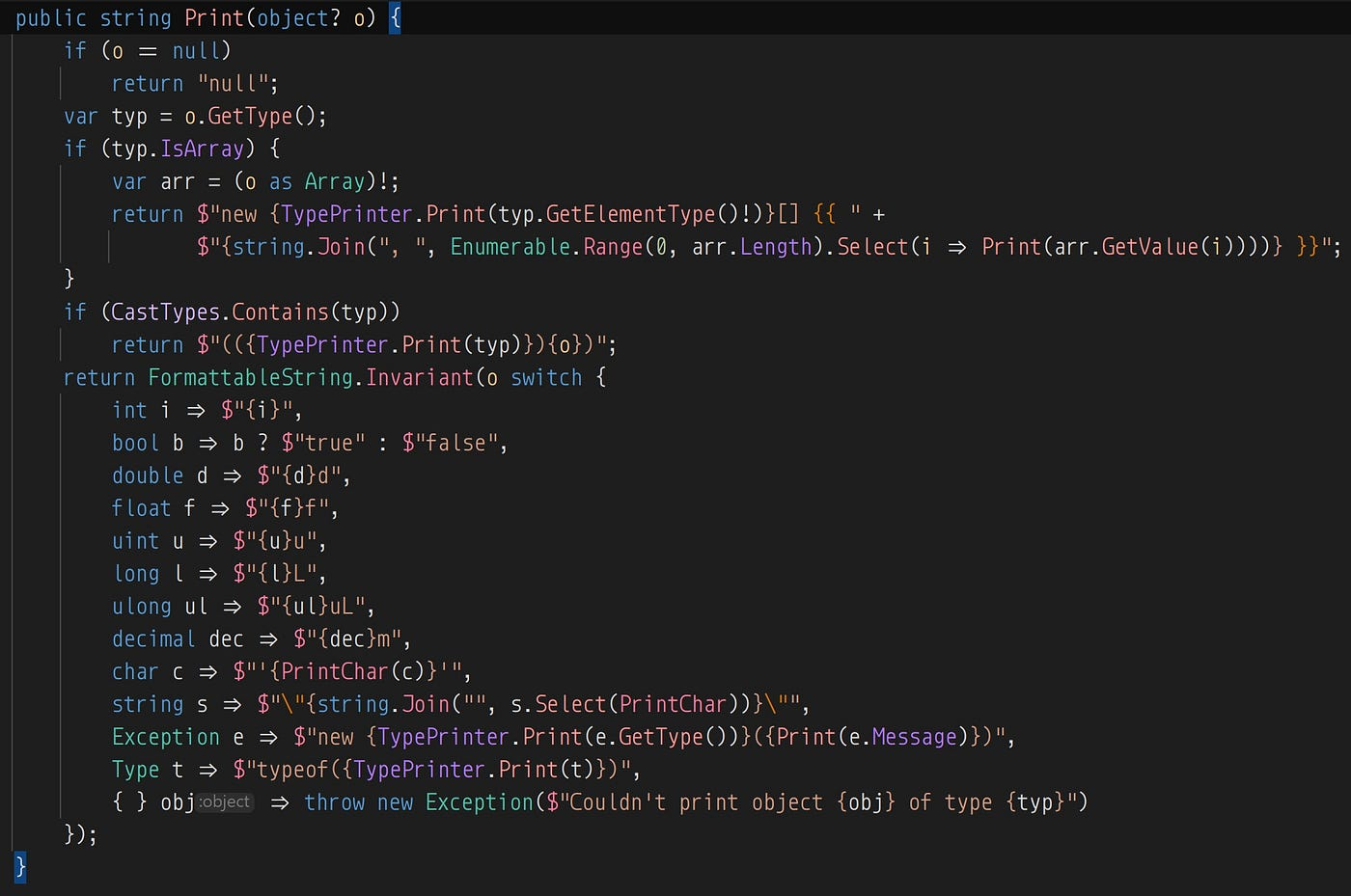Printing the contents of ConstantExpression. This is the first time I’ve ever thought that Python’s __repr__ might be useful.

If you’d like to take a look at the full code for LinearizeVisitor, you can find it here. And for good measure, here’s the code for the expression printer that I didn’t talk about. (The library this code is from is licensed under MIT.)

Thanks for reading. I’m planning to write more about fun technical problems in the near future, so look forward to it.

--

--

--

## More from Bagoum

Software engineer, epic gamer, and Touhou fangame developer.

Love podcasts or audiobooks? Learn on the go with our new app.

## IPFS Regions and Replications## What AWS CloudFormation Registry and CLI means for DevOps in the Cloud## Configure Apache as a forward proxy on Windows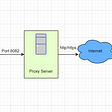## Creating a DevOps toolbox for Gitlab-CI## Intro to HTML-CSS: Part I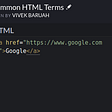## Setting up Python Environment on Top of Docker Container :## Bagoum

Software engineer, epic gamer, and Touhou fangame developer.

## How to stop forgetting to await an awaitable call## Providing a better experience for .NET developers with Caller Argument Expressions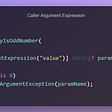## Enforcing a consistent code quality and style in your team-wide .NET projects## A JavaScript Rules Engine in .NET 6